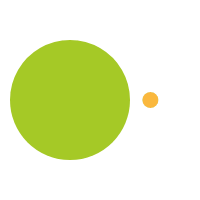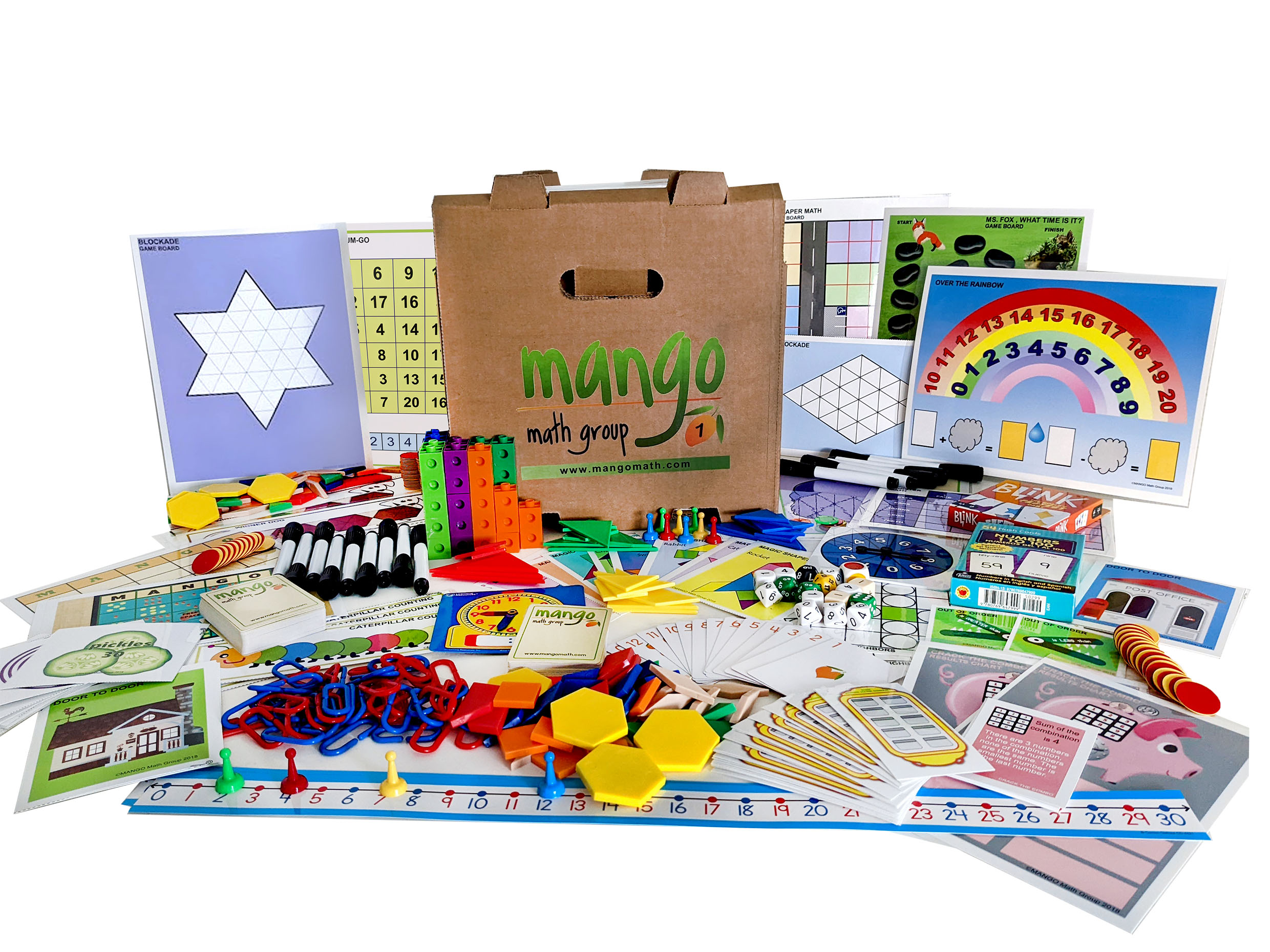#### Order Notice

As the economy opens back up, many of our suppliers are experiencing shortages of parts and raw materials, resulting in delays in shipments. As a result, we anticipate that it may take up to 2-4 weeks from the date we receive a purchase order for us to fulfill and ship any order for our products. We apologize for any inconvenience.

NEW!!

# First Grade Deluxe Math Kit

Our Strong Evidence Based MANGO Math First Grade Math Kit has creative math games and activities are exciting and fun! MANGO Math Deluxe First Grade Math Kit contains 20 separate skill building math lessons.

\$ 459.00 USD##### Product Details

MANGO Math’s First Grade Math Kit has creative math games and activities for math fun! MANGO Math Deluxe First Grade Math Kit contains 20 separate skill building math lessons.

Each individual lesson offers visual and kinetic representation of math concepts that develop strong math knowledge like:

• developing understanding of addition and subtraction
• expanding understanding of place value with groupings in tens and ones
• developing understanding of linear measurement
• expanding understanding of attributes of shapes and objects

Every lesson is based on a game or activity that fosters curiosity, discussion and growth through cooperation, collaboration, and creative thinking.

MANGO Math's Strong Evidence Based Kits come with individually packaged, Grab-n-Go lessons that includes instructions, reusable materials pre-packaged in are sealable pouch contained in an easy to carry crate that can be used year after year, providing long term savings.

A lesson can be used with by to 4 students, set up as math centers with each grade level math kit meeting the needs of 30+ students at a time.

Each MANGO Math grade level math kit is research-based and adheres to Common Core, TEKS and NCTM math standards.

Great to use in regular classrooms, resource rooms, after school programs, STEM programs, libraries, math centers, summer camps, learning cooperatives, math clubs, community centers, and at home.

#### All the supplies below are included in this pack:

• Spinners
• Numbered dice
• 10-sided dice
• 12-sided dice
• 20-sided dice
• Pattern block dice
• 3 colors dice
• Bi-colored counters
• Stacking counters
• Tangrams
• Geared time clock
• MANGO playing cards
• 1 – 30 number line
• Connecting cubes
• Pattern blocks
• Game pawns
• 1 – 100 numbered cards
• Game boards
• Custom game cards
• Laminated writing boards
• Dry erase markers
• Curriculum Guide

## First Grade Lessons with Standards

Door to Door – Measurement: Understand that the length measurement of an object is the number of same-size length units that span it with no gaps or overlays.

Ms. Fox, What Time Is It? – Measurement: Tell time in hours and half-hours using analog clocks.

Caterpillar Counting – Algebraic Thinking & Operation: Relative counting to addition and subtraction by counting on by 2 or add 2 and by counting on with 10s.

Crack the Combo – Algebraic Thinking & Operation: Using addition within 20 to solve word problems involving a situation of adding to, comparing.

Cue – Algebraic Thinking & Operation: Apply properties of operations as strategies to add and subtract.

Jump Off – Algebraic Thinking & Operation: Use addition and subtraction with 30 to solve situations of adding to, taking from, and comparing using drawings.

MANGO BINGO – Algebraic Thinking & Operation: Using addition or subtraction to solve problems by using objects to represent a number

Monster Math – Algebraic Thinking & Operation: Relate counting to addition by counting on 2 to add to 2 or counting on 3 to add 3.

Over the Rainbow – Algebraic Thinking & Operation: Determine the unknown whole number in an addition or subtraction equation relating three whole numbers.

Salute – Algebraic Thinking & Operation: Understand subtraction as an unknown-addend problem.

Tic-Sum-Toe – Algebraic Thinking & Operation: Adding three numbers together working on Commutative & Associative property of addition.

Winner Dog – Algebraic Thinking & Operation: Using addition to solve problems involving situations of putting together and comparing unknowns by using objects.

Number Neighbors – Number & Operation in Base Ten: Given a two-digit number, mentally find 10 more or 10 less than the number, without having to count.

Order Up – Number & Operation in Base Ten: Add or subtract multiples of 1– in the range of 10-90.

Out of Order – Number & Operation in Base Ten: Compare two-digit numbers based on meanings of the tens and one’s digit, recording the results of comparisons with the symbols >, =, <.

Roller Coaster Ride – Number & Operation in Base Ten: Add within 100, including adding a two-digit number and a one-digit number and adding a two-digit number and a multiple of ten.

Blockade – Geometry: Compose shapes to create a composite shape using; squares, triangles, rhombus, and trapezoids.

Magic Shapes – Geometry: Compose two-dimensional shapes to create a composite shape using, rectangles, squares, trapezoids, triangles.

Blink® - Odds & Order/Data: Organize, represent, and interpret data with up to three characteristics, number, shape, color

Skyscraper Math – Odds & Order/Data: Organize, represent, and interpret data with up to three categories; ask and answer questions about the total number of data points.

Width
13
in
Height
13
in
Length
19
in
Weight
20
lb